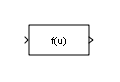Documentation

# Fcn

Apply specified expression to input

• Library:## Description

The Fcn block applies the specified mathematical expression to its input. The expression can include one or more of these components:

• `u` — The input to the block. If `u` is a vector, `u(i)` represents the `i`th element of the vector; `u(1)` or `u` alone represents the first element.

• Numeric constants.

• Arithmetic operators (+ - `*` / ^).

• Relational operators (`== != > < >= <=`) — The expression returns 1 if the relation is true; otherwise, it returns 0.

• Logical operators (&& || !) — The expression returns 1 if the relation is true; otherwise, it returns 0.

• Parentheses.

• Mathematical functions — `abs`, `acos`, `asin`, `atan`, `atan2`, `ceil`, `cos`, `cosh`, `exp`, `floor`, `hypot`, `log`, `log10`, `power`, `rem`, `sgn` (equivalent to `sign` in MATLAB®), `sin`, `sinh`, `sqrt`, `tan`, and `tanh`.

### Note

The Fcn block does not support `round` and `fix`. Use the Rounding Function block to apply these rounding modes.

• Workspace variables — Variable names that are not recognized in the preceding list of items are passed to MATLAB for evaluation. Matrix or vector elements must be specifically referenced (e.g., `A(1,1)` instead of `A` for the first element in the matrix).

The Fcn block observes the following rules of operator precedence:

1. `( )`

2. `^`

3. `+ - `(unary)

4. `!`

5. `* /`

6. `+ -`

7. `> < <= >=`

8. `== !=`

9. `&&`

10. `||`

The expression differs from a MATLAB expression in that the expression cannot perform matrix computations. Also, this block does not support the colon operator (:).

Block input can be a scalar or vector. The output is always a scalar. For vector output, consider using the Math Function block. If a block input is a vector and the function operates on input elements individually (for example, the `sin` function), the block operates on only the first vector element.

## Ports

### Input

expand all

The Fcn block accepts and outputs signals of type `single` or `double`.

Data Types: `single` | `double`

### Output

expand all

The Fcn block accepts and outputs signals of type `single` or `double`.

Data Types: `single` | `double`

## Parameters

expand all

Specify the mathematical expression to apply to the input. Expression components are listed above. The expression must be mathematically well-formed (uses matched parentheses, proper number of function arguments, and so on). The expression has restrictions on tunability (see Limitations).

#### Programmatic Use

 Block Parameter: `Expr` Type: character vector Value: mathematical expression Default: `'sin(u(1)*exp(2.3*(-u(2))))'`

### Note

This parameter is not visible in the block dialog box unless it is explicitly set to a value other than `-1`. To learn more, see Blocks for Which Sample Time Is Not Recommended.

## Block Characteristics

 Data Types `double` | `single` Direct Feedthrough `yes` Multidimensional Signals `no` Variable-Size Signals `no` Zero-Crossing Detection `no`